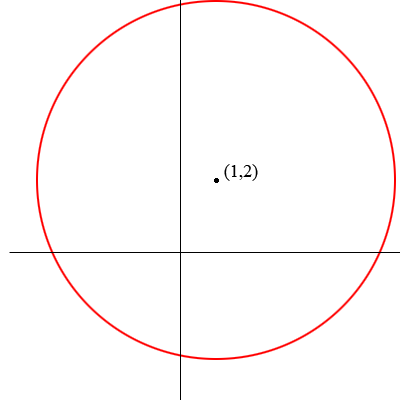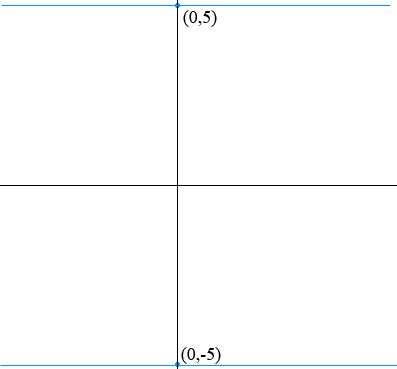SEARCH HOMEMath Central Quandaries & QueriesQuestion from Shaun: Find the points (x,y) in the plane that are 5 units from (1,2) and 5 units from y-axis. I am more interested in the approach, in general terms, than the numerical values. Thanks, ShaunShaun,

When I read this question I see two diagrams in my mind.

The points in the plane that are 5 units from (1,2) are the points on the circle with centre (1,2) and radius 5.The points that are 5 units from the x-axis are the points on the two lines 5 units above and 5 units below the x-axis.The points you want are on the the points where the two lines intersect the circle. Draw both diagrams on the same graph with the same scale and see if the diagram tells you how to find the coordinated of the points on intersection.

If you need more help write back,
PennyMath Central is supported by the University of Regina and The Pacific Institute for the Mathematical Sciences.